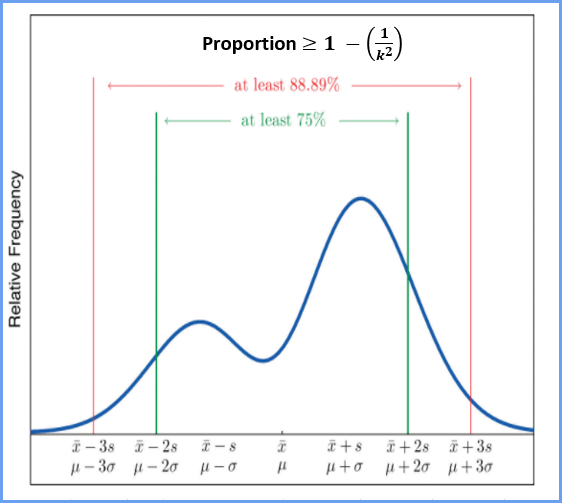# BUS 233## Chebyshev’s Theorem Excel Calculator

Chebyshev’s Excel Calculator Chebyshev’s Theorem can be used for any type of distribution, but if the problem says the distribution is “bell shaped,” use the Empirical Rule unless the problem specifically says to use Chebyshev’s. Note: an alternative spelling is Chebychev. Chebyshev’s only applies to problems where the K is > 1. In our MSL …

## Why is 2-tail p-value twice the 1-tail p-value?

Add Your Heading Text Here Why is the two-tail p-value always twice the one-tail p-value in a t-test? In the t-test output below, notice that the two-tail p-value is 0.66852 while the one-tail p-value is 0.33426. The two-tail p-value is twice the one-tail p-value. Why is this true? The two-tail p-value is defined as P …## Correlation and Chi-square Tests for Independence

The word “correlate” has several meanings, e.g. “If two or more facts, numbers, etc. correlate or are correlated, there is a relationship between them” (Cambridge Dictionary, n.d.) And “to show that a close connection exists between (two or more things)” (Merriam-Webster, 2019)  Also, “to show that two things are connected.” (Macmillan, n.d.) In statistics, we often …

## Empirical Rule and z-score Probability

Empirical Rule The Empirical Rule applies to a normal, bell-shaped curve than is symmetrical about the mean. It states that within one standard deviation of the mean (both left-side and right-side) there is about 68% of the data; within two standard deviations of the mean (both left-side and right-side) there is about 95% of the data; …